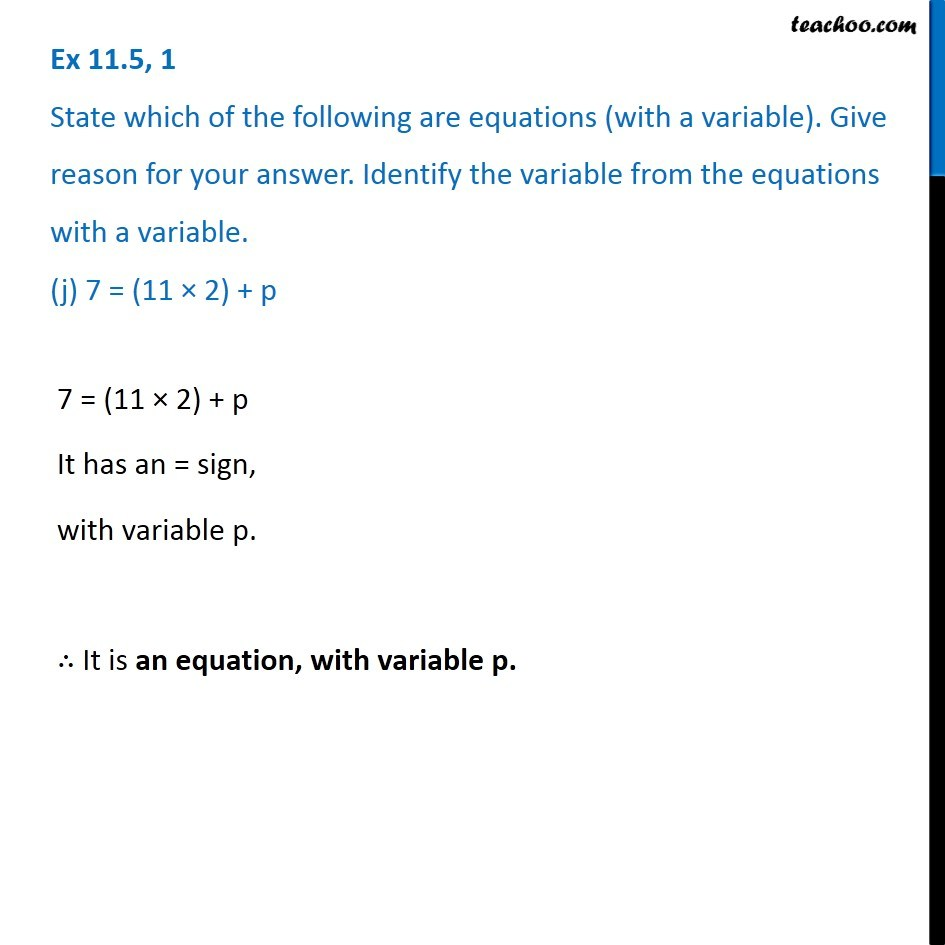Ex 11.5

Chapter 11 Class 6 Algebra
Serial order wiseIntroducing your new favourite teacher - Teachoo Black, at only ₹83 per month

### Transcript

Ex 11.5, 1 State which of the following are equations (with a variable). Give reason for your answer. Identify the variable from the equations with a variable. (j) 7 = (11 × 2) + pEx 11.5, 1 State which of the following are equations (with a variable). Give reason for your answer. Identify the variable from the equations with a variable. (j) 7 = (11 × 2) + p